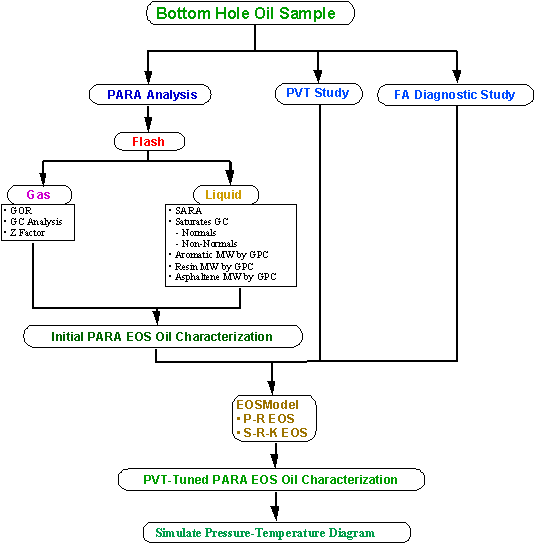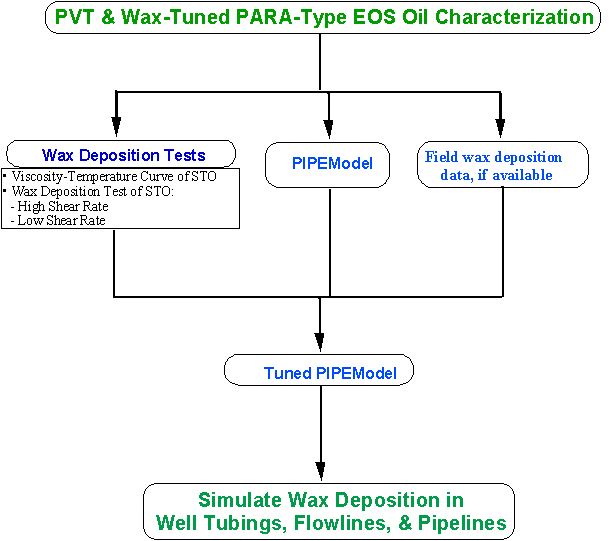Wax Deposition Study

Objective:

A Wax Deposition Study's purpose is to determine the wax deposition rate during production of a hydrocarbon fluid in a given facility layout. The wax deposition rate from the fluid is first determined in the lab equipment. Then, the wax deposition rate of the lab system is simulated in order to tune a multi-phase wax deposition simulator, like AsphWax's PIPEModelR. Finally, the wax deposition rate of the field system is simulated with the tuned PIPEModelR. The wax deposition rate is utilized to evaluate the need for insulation, pigging, and chemical treatments.

Description

A Wax Deposition Study is a continuation of a Wax Phase Behavior Study and it involves a sequence of tests and computer simulation leading to determination of the wax deposition rate. The flowcharts below give a summary of the steps required to:

• Obtain representative bottom-hole fluid samples

• Perform PARA (Paraffin-Aromatic-Resin-Asphaltene) Analysis, PVT Study, and Flow Assurance Diagnostic Study

• Develop a PVT-tuned PARA EOS fluid characterization

• Simulate the fluid's P-T Diagram

• Tune wax phase behavior model

• Simulate the fluid's WDE

• Obtain laboratory wax deposition rate at two or three (preferred) shear rates, bounding the field-expected shear rates.

• Tune multiphase wax deposition simulator to the lab data

• Simulate the field wax deposition rate